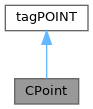ReactOS 0.4.15-dev-6712-g46b4b55
CPoint Class Reference

`#include <atltypes.h>`

Inheritance diagram for CPoint:[legend]
Collaboration diagram for CPoint:[legend]

## Public Member Functions

CPoint () throw ()

CPoint (int initX, int initY) throw ()

CPoint (POINT initPt) throw ()

CPoint (SIZE initSize) throw ()

CPoint (LPARAM dwPoint) throw ()

void Offset (int xOffset, int yOffset) throw ()

void Offset (POINT point) throw ()

void Offset (SIZE size) throw ()

BOOL operator== (POINT point) const throw ()

BOOL operator!= (POINT point) const throw ()

void operator+= (SIZE size) throw ()

void operator+= (POINT point) throw ()

void operator-= (SIZE size) throw ()

void operator-= (POINT point) throw ()

CPoint operator+ (SIZE size) const throw ()

CPoint operator+ (POINT point) const throw ()

CRect operator+ (const RECT *lpRect) const throw ()

CSize operator- (POINT point) const throw ()

CPoint operator- (SIZE size) const throw ()

CRect operator- (const RECT *lpRect) const throw ()

CPoint operator- () const throw ()Public Attributes inherited from tagPOINT
long x

long y

LONG x

LONG y

## Detailed Description

Definition at line 28 of file atltypes.h.

## ◆ CPoint() [1/5]

 CPoint::CPoint ( ) throw ( )
inline

Definition at line 32 of file atltypes.h.

33 {
34 x = y = 0;
35 }
GLint GLint GLint GLint GLint x
Definition: gl.h:1548
GLint GLint GLint GLint GLint GLint y
Definition: gl.h:1548

Referenced by operator+(), and operator-().

## ◆ CPoint() [2/5]

 CPoint::CPoint ( int initX, int initY ) throw ( )
inline

Definition at line 37 of file atltypes.h.

38 {
39 x = initX;
40 y = initY;
41 }

## ◆ CPoint() [3/5]

 CPoint::CPoint ( POINT initPt ) throw ( )
inline

Definition at line 43 of file atltypes.h.

44 {
45 *((POINT*)this) = initPt;
46 }

## ◆ CPoint() [4/5]

 CPoint::CPoint ( SIZE initSize ) throw ( )
inline

Definition at line 48 of file atltypes.h.

49 {
50 *((SIZE*)this) = initSize;
51 }

## ◆ CPoint() [5/5]

 CPoint::CPoint ( LPARAM dwPoint ) throw ( )
inline

Definition at line 53 of file atltypes.h.

54 {
55 x = LOWORD(dwPoint);
56 y = HIWORD(dwPoint);
57 }
#define LOWORD(l)
Definition: pedump.c:82
#define HIWORD(l)
Definition: typedefs.h:247

## ◆ Offset() [1/3]

 void CPoint::Offset ( int xOffset, int yOffset ) throw ( )
inline

Definition at line 59 of file atltypes.h.

60 {
61 x += xOffset;
62 y += yOffset;
63 }
int yOffset
Definition: appswitch.c:59
int xOffset
Definition: appswitch.c:59

## ◆ Offset() [2/3]

 void CPoint::Offset ( POINT point ) throw ( )
inline

Definition at line 65 of file atltypes.h.

66 {
68 }
POINTL point
Definition: edittest.c:50
_In_ ULONG _In_ ULONG Offset
Definition: ntddpcm.h:101
LONG y
Definition: windef.h:330
LONG x
Definition: windef.h:329

## ◆ Offset() [3/3]

 void CPoint::Offset ( SIZE size ) throw ( )
inline

Definition at line 70 of file atltypes.h.

71 {
72 Offset(size.cx, size.cy);
73 }
GLsizeiptr size
Definition: glext.h:5919

## ◆ operator!=()

 BOOL CPoint::operator!= ( POINT point ) const throw ( )
inline

Definition at line 80 of file atltypes.h.

81 {
82 return !(*this == point);
83 }

## ◆ operator+() [1/3]

 CRect CPoint::operator+ ( const RECT * lpRect ) const throw ( )
inline

Definition at line 542 of file atltypes.h.

543{
544 CRect r(lpRect);
545 r += *this;
546 return r;
547}
GLdouble GLdouble GLdouble r
Definition: gl.h:2055

## ◆ operator+() [2/3]

 CPoint CPoint::operator+ ( POINT point ) const throw ( )
inline

Definition at line 110 of file atltypes.h.

111 {
112 return CPoint(x + point.x, y + point.y);
113 }
CPoint()
Definition: atltypes.h:32

## ◆ operator+() [3/3]

 CPoint CPoint::operator+ ( SIZE size ) const throw ( )
inline

Definition at line 105 of file atltypes.h.

106 {
107 return CPoint(x + size.cx, y + size.cy);
108 }

## ◆ operator+=() [1/2]

 void CPoint::operator+= ( POINT point ) throw ( )
inline

Definition at line 90 of file atltypes.h.

91 {
93 }

## ◆ operator+=() [2/2]

 void CPoint::operator+= ( SIZE size ) throw ( )
inline

Definition at line 85 of file atltypes.h.

86 {
87 Offset(size);
88 }

## ◆ operator-() [1/4]

 CPoint CPoint::operator- ( ) const throw ( )
inline

Definition at line 126 of file atltypes.h.

127 {
128 return CPoint(-x, -y);
129 }

## ◆ operator-() [2/4]

 CRect CPoint::operator- ( const RECT * lpRect ) const throw ( )
inline

Definition at line 549 of file atltypes.h.

550{
551 CRect r(lpRect);
552 r -= *this;
553 return r;
554}

## ◆ operator-() [3/4]

 CSize CPoint::operator- ( POINT point ) const throw ( )
inline

Definition at line 215 of file atltypes.h.

216{
217 return CSize(x - point.x, y - point.y);
218}

## ◆ operator-() [4/4]

 CPoint CPoint::operator- ( SIZE size ) const throw ( )
inline

Definition at line 119 of file atltypes.h.

120 {
121 return CPoint(x - size.cx, y - size.cy);
122 }

## ◆ operator-=() [1/2]

 void CPoint::operator-= ( POINT point ) throw ( )
inline

Definition at line 100 of file atltypes.h.

101 {
102 Offset(-point.x, -point.y);
103 }

## ◆ operator-=() [2/2]

 void CPoint::operator-= ( SIZE size ) throw ( )
inline

Definition at line 95 of file atltypes.h.

96 {
97 Offset(-size.cx, -size.cy);
98 }

## ◆ operator==()

 BOOL CPoint::operator== ( POINT point ) const throw ( )
inline

Definition at line 75 of file atltypes.h.

76 {
77 return (x == point.x && y == point.y);
78 }

The documentation for this class was generated from the following file: